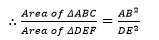Guru

# Let ΔABC ~ ΔDEF and their areas be, respectively, 64 cm2 and 121 cm2. If EF = 15.4 cm, find BC. Q.1

• 0

How i solve the important question from the chapter Triangles of exercise 6.4, It is very important for the board exam so please help to solve this problem Let ΔABC ~ ΔDEF and their areas be, respectively, 64 cm2 and 121 cm2. If EF = 15.4 cm, find BC.

Share

1. Given, ΔABC ~ ΔDEF,

Area of ΔABC = 64 cm2

Area of ΔDEF = 121 cm2

EF = 15.4 cmAs we know, if two triangles are similar, ratio of their areas are equal to the square of the ratio of their corresponding sides,

= AC2/DF2 = BC2/EF2

∴ 64/121 = BC2/EF2

⇒ (8/11)2 = (BC/15.4)2

⇒ 8/11 = BC/15.4

⇒ BC = 8×15.4/11

⇒ BC = 8 × 1.4

⇒ BC = 11.2 cm

• 0